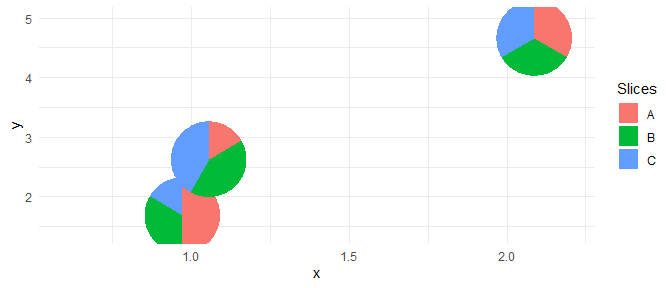# Unusual Situations

This vignette shows examples of potential unusual situations that can be encountered when using the PieGlyph package and how they are handled within the package

library(PieGlyph)
library(ggplot2)
library(dplyr)
library(tidyr)

### Missing values for a particular attribute

When data is missing, it is common to see a missing value for a particular attribute in an observation.

plot_data <- data.frame(x = 1:4,
y = c(3,1,4,2),
A = c(5, 2, NA, 3),
B = c(NA, 2, 3, NA),
C = c(7, NA, NA, 3))
plot_data
#>   x y  A  B  C
#> 1 1 3  5 NA  7
#> 2 2 1  2  2 NA
#> 3 3 4 NA  3 NA
#> 4 4 2  3 NA  3

The package handles this situation be replacing the any NA values with 0 in the data. The user is notified about this via a warning. This warning can be silenced by specifying na.rm as TRUE

ggplot()+
geom_pie_glyph(aes(x = x, y = y),
slices = c('A','B','C'),
data = plot_data,
theme_minimal()+
xlim(0.5,4.5)+
ylim(0.5,4.5)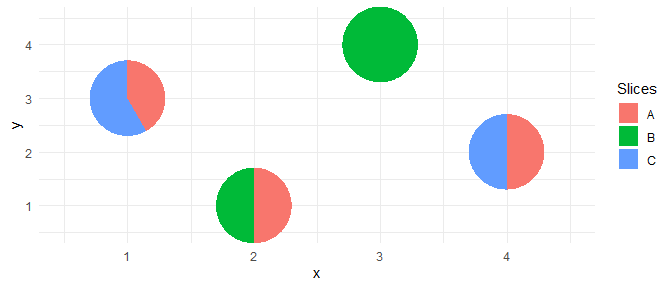### Missing values for all attributes

It is also possible that all attributes will be missing for an observation.

plot_data <- data.frame(x = 1:4,
y = c(3,1,4,2),
A = c(5, 2, NA, 1),
B = c(2, 2, NA, 5),
C = c(7, 3, NA, 3))
#>   x y  A  B  C
#> 1 1 3  5  2  7
#> 2 2 1  2  2  3
#> 3 3 4 NA NA NA
#> 4 4 2  1  5  3

The package handles this situation by dropping the observation that has all attributes as missing. The user is notified about this via a warning. This warning can be silenced by specifying na.rm as TRUE

ggplot(data = plot_data)+
geom_pie_glyph(aes(x = x, y = y),
slices = c('A','B','C'),
theme_minimal()+
xlim(0.5,4.5)+
ylim(0.5,4.5)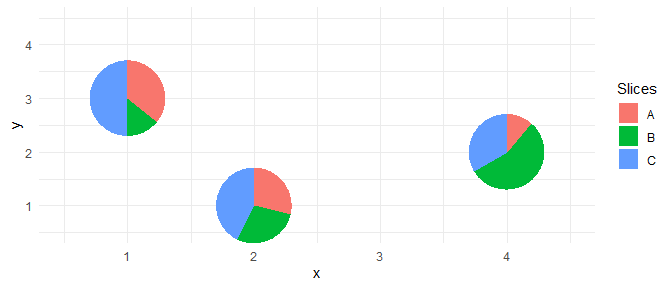### Negative values for attributes

Negative values don’t make sense in the context of pie-charts and are not supported by the PieGlyph package. If a user tries to use the package with data that includes negative values for attributes, they will get an error message.

plot_data <- data.frame(x = 1:4,
y = c(3,1,4,2),
A = c(5, -2, 3, 3),
B = c(2, 2, 0, 0),
C = c(-7, 1, 3, -3))
plot_data
#>   x y  A B  C
#> 1 1 3  5 2 -7
#> 2 2 1 -2 2  1
#> 3 3 4  3 0  3
#> 4 4 2  3 0 -3
ggplot()+
geom_pie_glyph(aes(x = x, y = y),
slices = c('A','B','C'),
data = plot_data)
#> Error in geom_pie_glyph():
#> ! Problem while converting geom to grob.
#> ℹ Error occurred in the 1st layer.
#> Caused by error in draw_panel():
#> ! Data contains negative values. Remove them before plotting.

### Identical x and y values for different pie-glyphs

If two or more pie-glyphs have identical values for the x and y coordinates, it can cause problems while creating the glyphs as the slices within each pie are grouped based on the x and y coordinates.

As an example, the plot generated for the following dataset should show two pie-glyphs (one for each system) showing the proportions of the three attributes. Although the two pie-glyphs would be overlapped over one another and only one would be visible.

dummy_data <- data.frame(system = rep(paste0('S', 1:3), each = 3),
x = c(1,1,1,1,1,1,2,2,2),
y = c(2,2,2,2,2,2,4,4,4),
attribute = rep(c('A','B','C'), times = 3),
value = c(30,20,10, 20,50,50, 10,10,10))
dummy_data
#>   system x y attribute value
#> 1     S1 1 2         A    30
#> 2     S1 1 2         B    20
#> 3     S1 1 2         C    10
#> 4     S2 1 2         A    20
#> 5     S2 1 2         B    50
#> 6     S2 1 2         C    50
#> 7     S3 2 4         A    10
#> 8     S3 2 4         B    10
#> 9     S3 2 4         C    10

The expected plot is as follows

ggplot(data = dummy_data[4:9,])+
geom_pie_glyph(aes(x = x, y = y),
slices = 'attribute', values = 'value',
theme_minimal()+
xlim(0.5, 2.5)+
ylim(1.5, 4.5)+
labs(title = 'Expected Plot')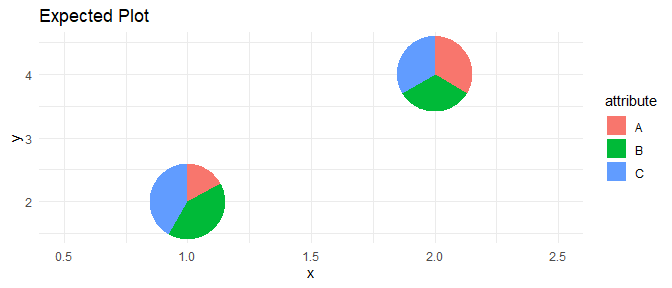However the plot produced is different and the attributes between the two system get combined into a single pie-glyph.

ggplot(data = dummy_data)+
geom_pie_glyph(aes(x = x, y = y),
slices = 'attribute', values = 'value',
theme_minimal()+
xlim(0.5, 2.5)+
ylim(1.5, 4.5)+
labs(title = 'Generated Plot')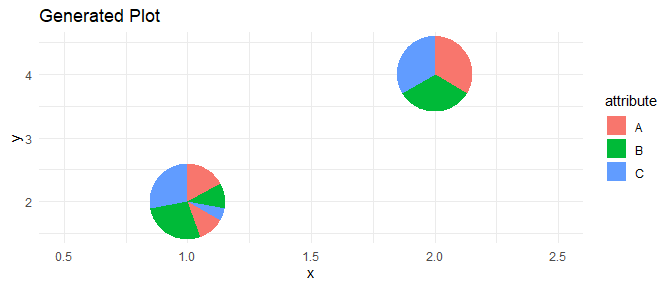As described before this is because the slices of the pie-glyph are grouped on the basis of the x and y coordinates of the pie-glyph and hence if two glyphs have the same x and y coordinates, their glyphs get combined together.

This problem arises only in situations when the data is presented in the long-format (attributes stacked in one column). The package warns the user if this issue arises.

ggplot(data = dummy_data)+
geom_pie_glyph(aes(x = x, y = y),
slices = 'attribute', values = 'value',
xlim(0.5, 2.5)+
ylim(1.5, 4.5)+
theme_minimal()
#> Warning in setup_data(...): Some pie-glyphs have identical x and y
#> coordinates. This can cause issues when creating the glyphs. Consider adding
#> a pie_group variable to distinguish individual glyphs from one another. See
#> vignette("unusual-situations") for more information.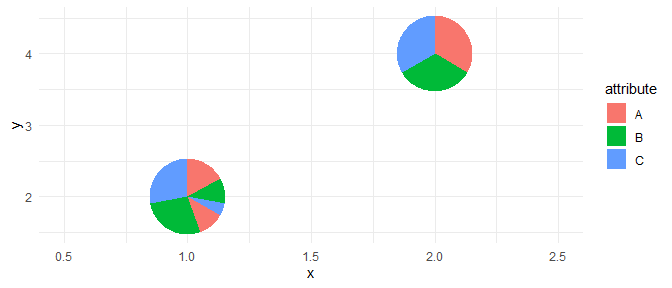The solution here is to manually specify the pie_group aesthetic which groups the attributes for one pie-glyph together

ggplot(data = dummy_data)+
geom_pie_glyph(aes(x = x, y = y, pie_group = system),
slices = 'attribute', values = 'value',
xlim(0.5, 2.5)+
ylim(1.5, 4.5)+
theme_minimal()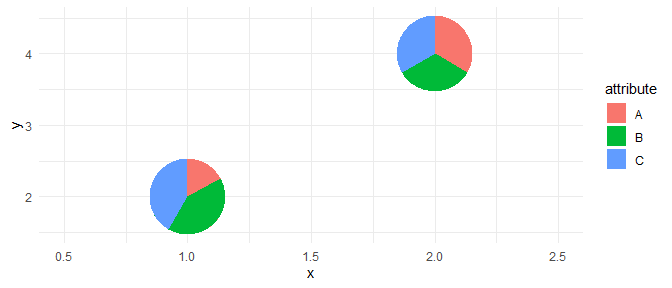However a grouping variable might not always be present in the data nor would it be easy to manually create one. Hence the easiest fix would be to simply convert the data into wide-format (unstack the attributes into separate columns) and let the package handle the grouping of the pie-glyphs.

dummy_data_wide <- dummy_data %>%
pivot_wider(names_from = 'attribute', values_from = 'value')

#> # A tibble: 3 × 6
#>   system     x     y     A     B     C
#>   <chr>  <dbl> <dbl> <dbl> <dbl> <dbl>
#> 1 S1         1     2    30    20    10
#> 2 S2         1     2    20    50    50
#> 3 S3         2     4    10    10    10

ggplot(data = dummy_data_wide)+
geom_pie_glyph(aes(x = x, y = y),
slices = c('A','B','C'),
xlim(0.5, 2.5)+
ylim(1.5, 4.5)+
theme_minimal()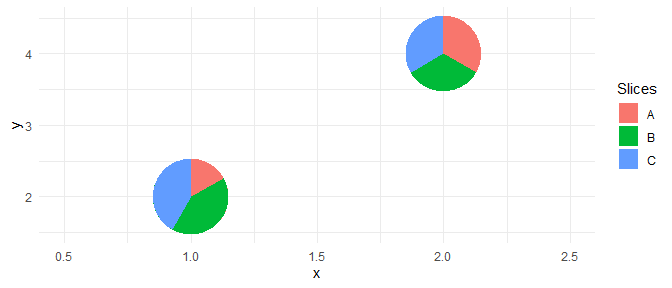Finally, we could jitter the pie-glyphs to view all the glyphs

ggplot(data = dummy_data_wide)+
geom_pie_glyph(aes(x = x, y = y),
slices = c('A','B','C'),
theme_minimal()